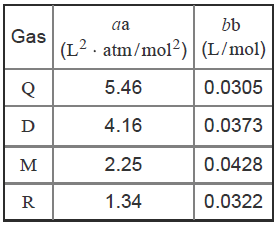# Problem: To prevent tank rupture during deep-space travel, an engineering team is studying the effect of temperature on gases confined to small volumes. What is the pressure of 2.80 mol of gas D measured at 251 °C in a 1.75-L container assuming real behavior?Express your answer with the appropriate units.You may be familiar with the ideal gas equation,PV=nRTwhere n is the number of moles, P is the pressure in atmospheres, V is the volume in liters, and R is equal to 0.08206 L ⋅ atm/(mol ⋅ K).Real gases, especially under extremes of temperature and pressure, deviate from ideal behavior due to intermolecular forces and the volume occupied by the gas molecules themselves. Correction factors can be applied to the ideal gas equation to arrive at the van der Waals equation,P=nRTV-nb-n2aV2where a and b are van der Waals constants for the particular gas. The term nb corrects the volume to account for the size of the molecules and the term n2a/V2 corrects the pressure for intermolecular attraction.van der Waals constants for hypothetical gases

###### FREE Expert SolutionView Complete Written Solution
###### Problem Details

To prevent tank rupture during deep-space travel, an engineering team is studying the effect of temperature on gases confined to small volumes. What is the pressure of 2.80 mol of gas D measured at 251 °C in a 1.75-L container assuming real behavior?

You may be familiar with the ideal gas equation,

$\mathbf{PV}\mathbf{=}\mathbf{nRT}$

where n is the number of moles, P is the pressure in atmospheres, V is the volume in liters, and R is equal to 0.08206 L ⋅ atm/(mol ⋅ K).

Real gases, especially under extremes of temperature and pressure, deviate from ideal behavior due to intermolecular forces and the volume occupied by the gas molecules themselves. Correction factors can be applied to the ideal gas equation to arrive at the van der Waals equation,

$\mathbf{P}\mathbf{=}\frac{\mathbf{nRT}}{\mathbf{V}\mathbf{-}\mathbf{nb}}\mathbf{-}\frac{{\mathbf{n}}^{\mathbf{2}}\mathbf{a}}{{\mathbf{V}}^{\mathbf{2}}}$

where a and b are van der Waals constants for the particular gas. The term nb corrects the volume to account for the size of the molecules and the term n2a/V2 corrects the pressure for intermolecular attraction.

van der Waals constants for hypothetical gases